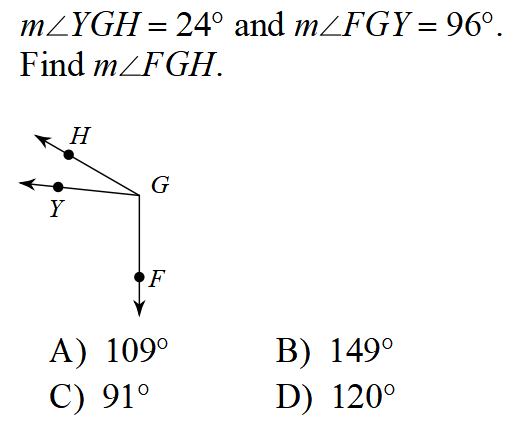Geo activity 1-3 Practice
7. Angle X and Y are complementary. Angle X is 3 times larger than Y. What's the measure of Y? *
0 points
9.Find the measure of each numbered angle *
1 point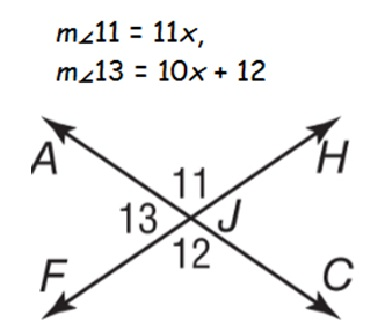Last Name *
4. *
1 point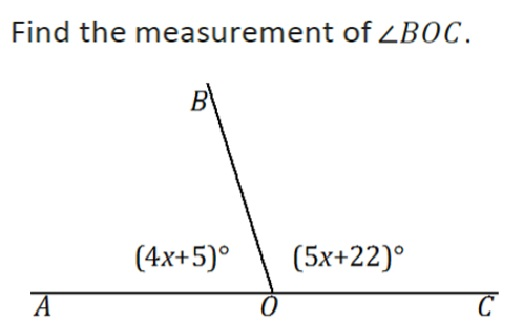Read carefully, setup L + L = B, and find x. *
1 point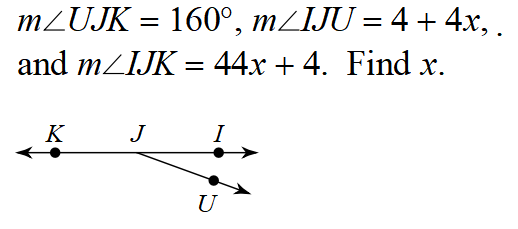FIND THE MEASURE OF <CBD!!!!!!!!!! *
1 point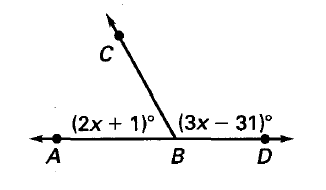1. Find the measure of angle C *
1 pointDistance Formula *
1 point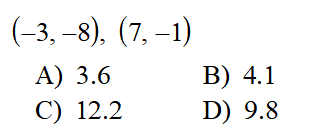Solve *
1 point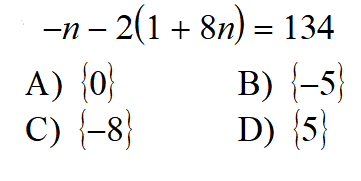These two angles are non-adjacent complementary angles. find x. *
1 point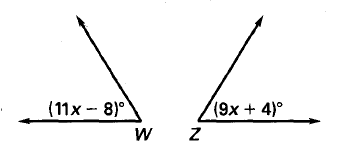Period *
Solve for x *
1 point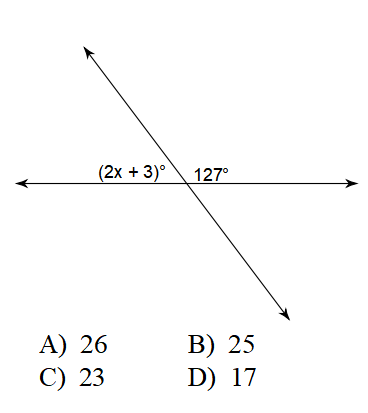2. What is measure of angle w? *
1 point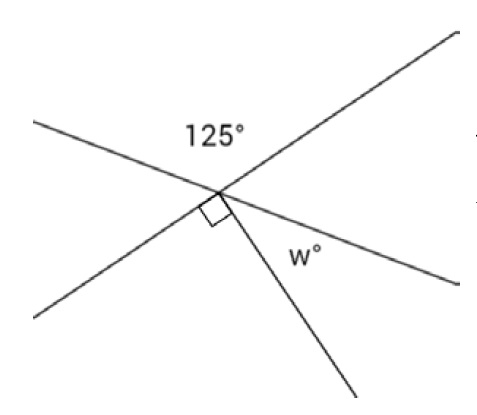5. *
1 point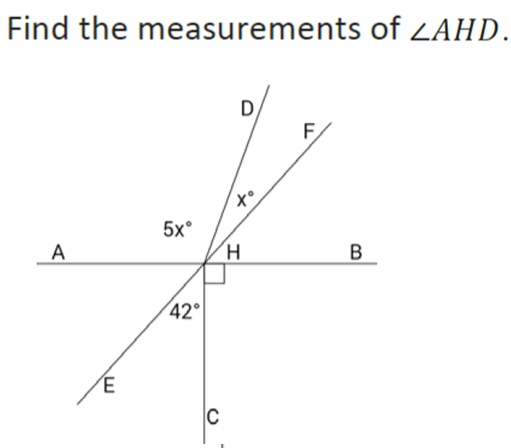8. Two angles are complementary. One angle is 18 less than the other. Find the measure of the smaller angle. *
1 point
6. *
1 point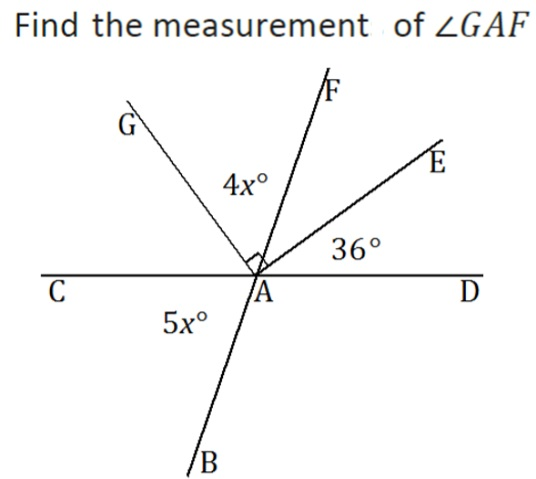Distance formula *
1 point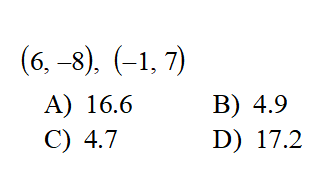Find the measure of <BED if <AEB = 16 degrees and <BEC = 77 degrees. *
1 point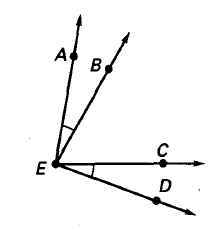solve for x *
1 point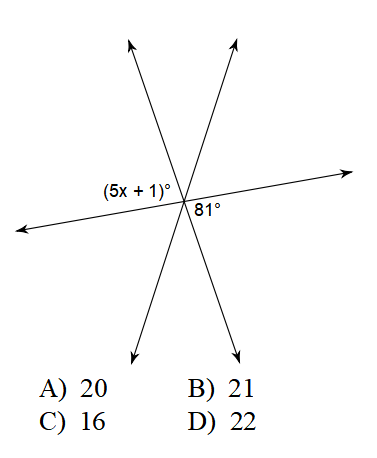Find measure of angle x *
1 point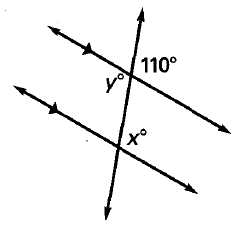3. *
1 point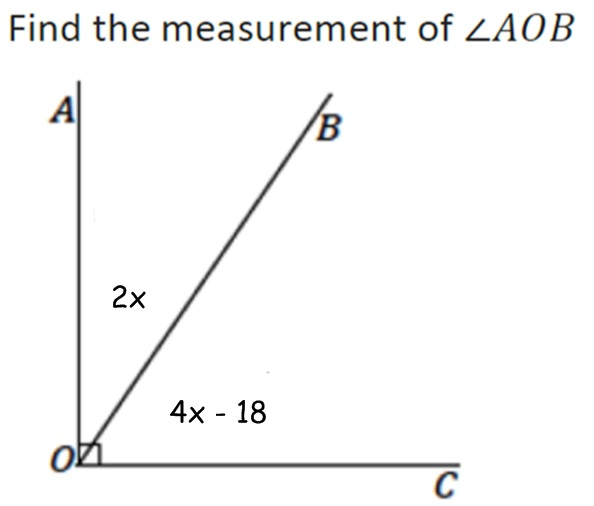solve *
1 point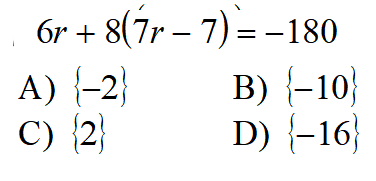Read carefully and find missing angle *
1 point